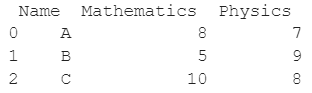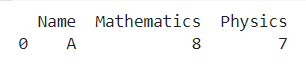# How Do I Select Rows From A DataFrame Based On Column Values?

A DataFrame is the primary data structure of the Pandas library and is commonly used for storing and working with tabular data. A common operation that could be performed on such data is to select rows from a DataFrame based on column values in order to extract more information from the DataFrame.

To start working with Pandas, we first need to import this statement in the Python code :

### Import Pandas in Python

``import pandas as pd``

## Running Example

Let us understand this operation with the help of an example. Consider the following DataFrame containing 3 students with names A, B, and C and their corresponding marks (out of 10) for two subjects, Mathematics and Physics.Python code snippet for generating the above DataFrame :

### Python 3 Code :

``````# Importing pandas
import pandas as pd

# Dictionary for our data
data = {'Name' : ['A', 'B', 'C'], 'Mathematics' : [8, 5, 10], 'Physics' : [7, 9, 8]}

# DataFrame for the dictionary
df = pd.DataFrame(data)

# Printing the DataFrame
print(df)``````

Here, data is a dictionary we created to initialize the DataFrame. For this, we use the DataFrame() function of the Pandas library which takes the dictionary as an argument and returns the required DataFrame.

Now, let’s say we need to select only the rows with physics marks equal to 7. For our dataframe, this would be the first row. The resulting DataFrame would look like this :Let us look at different ways of performing this operation on a given DataFrame :

## Select Rows From A DataFrame Using boolean masking

In this code example, we use boolean expressions to decide which rows will be selected and which ones won’t be selected.

Here, the selection criteria are that the marks in physics should be equal to 7. Here, df[‘Physics’] is used to access the column with the label Physics in the DataFrame. The changes are not made in place so we need to reassign the dataframe.

Let us look at the Python code and corresponding output for this method.

``````# Importing pandas
import pandas as pd

# Dictionary for our data
data = {'Name' : ['A', 'B', 'C'], 'Mathematics' : [8, 5, 10], 'Physics' : [7, 9, 8]}

# DataFrame for the dictionary
df = pd.DataFrame(data)

# Performing the operation
df = df[df['Physics'] == 7]

# Printing the updated DataFrame
print(df)``````

Output :Another way to perform the same operation would be to use df.Physics to access the column labeled as Physics instead of using df[‘Physics’]. The changes are not made in place so we need to reassign the dataframe.

Let us look at the Python code and corresponding output for this method.

``````# Importing pandas
import pandas as pd

# Dictionary for our data
data = {'Name' : ['A', 'B', 'C'], 'Mathematics' : [8, 5, 10], 'Physics' : [7, 9, 8]}

# DataFrame for the dictionary
df = pd.DataFrame(data)

# Performing the operation
df = df[df.Physics == 7]

# Printing the updated DataFrame
print(df)``````

Output## Select Rows From A DataFrame Using the dataframe.loc property

This method is an alternative method to the previous one. In this method, we use the dataframe.loc property to select specific rows based on certain values in columns.

Here, the selection criteria are that marks in physics should be equal to 7. Here, df[‘Physics’] is used to access the column with the label Physics in the DataFrame. The changes are not made in place so we need to reassign the dataframe.

Let us look at the Python code and corresponding output for this method:

``````# Importing pandas
import pandas as pd

# Dictionary for our data
data = {'Name' : ['A', 'B', 'C'], 'Mathematics' : [8, 5, 10], 'Physics' : [7, 9, 8]}

# DataFrame for the dictionary
df = pd.DataFrame(data)

# Performing the operation
df = df.loc[df['Physics'] == 7]

# Printing the updated DataFrame
print(df)``````

OutputAnother way to perform the same operation would be to use df.Physics to access the column labeled as Physics instead of using df[‘Physics’]. The changes are not made in place so we need to reassign the dataframe. Let us look at the Python code and corresponding output for this method -

``````# Importing pandas
import pandas as pd

# Dictionary for our data
data = {'Name' : ['A', 'B', 'C'], 'Mathematics' : [8, 5, 10], 'Physics' : [7, 9, 8]}

# DataFrame for the dictionary
df = pd.DataFrame(data)

# Performing the operation
df = df.loc[df.Physics == 7]

# Printing the updated DataFrame
print(df)``````

Output## Select Rows From A DataFrame Using the dataframe.query() function

This method is an alternative method to the previous ones. In this method, we use the dataframe.query() function to select specific rows based on certain values in columns.

Here, the selection criteria are that marks in physics should be equal to 7. Therefore, the required query is Physics == 7. The changes are not made in place so we need to reassign the dataframe.

Let us look at the Python code and corresponding output for this method.

``````# Importing pandas
import pandas as pd

# Dictionary for our data
data = {'Name' : ['A', 'B', 'C'], 'Mathematics' : [8, 5, 10], 'Physics' : [7, 9, 8]}

# DataFrame for the dictionary
df = pd.DataFrame(data)

# Performing the operation
df = df.query('Physics == 7')

# Printing the updated DataFrame
print(df)``````

Output## Conclusion

In this topic, we have learned how to select rows from a DataFrame based on column values in an existing Pandas DataFrame, following a running example of test scores of students in different subjects, thus giving us an intuition of how this concept could be applied in real-world situations. Feel free to reach out to info.javaexercise@gmail.com in case of any suggestions

Trending